# Samacheer Kalvi 11th Economics Solutions Chapter 3 Production Analysis

Guys who are planning to learn the fundamentals of Economics Solutions can avail the handy study material Samacheer Kalvi Class 11th Economics Solutions Chapter 3 Production Analysis Questions and Answers here. Refer to the Samacheer Kalvi Economics Solutions for Class 11th PDF available and score better grades. Simply click on the quick links available for Samacheer Kalvi 11th Economics Solutions Chapter 3 Production Analysis and prepare all the concepts in it effectively. Take your preparation to the next level by availing the Tamilnadu State Board Solutions for Class 11th Economics Solutions Chapter 3 Production Analysis prepared by subject experts.

## Tamilnadu Samacheer Kalvi 11th Economics Solutions Chapter 3 Production Analysis

Do you feel the concept of Economics Solutions difficult to understand? Not anymore with our Samacheer Kalvi for Class 11th Economics Solutions Chapter 3 Production Analysis Questions and Answers. All the Solutions are given to you with detailed explanation and you can enhance your subject knowledge. Use the Samacheer Kalvi Textbook Chapter 3 Production Analysis Solutions PDF free of cost and prepare whenever you want.

### Samacheer Kalvi 11th Economics Production Analysis Text Book Back Questions and Answers

Part – A

Multiple Choice Questions

11th Economics Chapter 3 Book Back Answers Question 1.
The primary factors of production are:
(a) Labour and Organisation
(b) Labour and Capital
(c) Land and Capital
(d) Land and Labour.
(d) Land and Labour.

Samacheer Kalvi Guru 11th Economics Question 2.
The man-made physical goods used to produce other goods and services are referred to as …………………………
(a) Land
(b) Labour
(c) Capital
(d) Organization
(c) Capital

Economics Class 11 Chapter 3 Question Answers Question 4.
Which factor is called the changing agent of society?
(a) Labourer
(b) Land
(c) Organizer
(d) Capital
(c) Organizer

Samacheer Kalvi Guru 11 Economics Question 5.
Who said, that one of the key of an entrepreneur is “uncertainty-bearing”.
(a) J.B.Clark
(b) Schumpeter
(c) Knight
(c) Knight

Samacheer Kalvi 11th Economics Solutions Question 6.
The functional relationship between “inputs” and “outputs” is called………………………..
(a) Consumption Function
(b) Production Function
(c) Savings Function
(d) Investment Function
(b) Production Function

Samacheer Kalvi Economics 11th Question 7.
In a firm 5 units of factors produce 24 units of the product. When the number of factor increases by one, the production increases to 30 units. Calculate the Average Product.
(a) 30
(b) 6
(c) 5
(d) 24
(c) 5

Samacheer Kalvi Class 11 Economics Solutions Question 8.
The short-run production is studied through ………………………
(a) The Laws of Returns to Scale
(b) The Law of Variable Proportions
(c) Iso – quants
(d) Law of Demand
(b) The Law of Variable Proportions

11 Th Samacheer Kalvi Economics Question 9.
The long-run production function is explained by
(a) Law of Demand
(b) Law of Supply
(c) Returns to Scale
(d) Law of Variable Proportions
(c) Returns to Scale

Economics Chapter 3 Question 10.
An Iso – quant curve is also known as …………………………
(a) Inelastic Supply curve
(b) Inelastic Demand Curve
(c) Equi – marginal Utility
(d) Equal Product Curve
(d) Equal Product Curve

Production Function Questions And Answers Question 11.
Mention the economies reaped from inside the firm
(a) Financial
(b) Technical
(c) Managerial
(d) All of the above
(d) All of the above

Production Function Problems And Solutions Pdf Question 12.
Cobb – Douglas production function assumes ………………………….
(a) Increasing returns to scale
(b) Diminishing returns to scale
(c) Constant returns to scale
(d) All of the above
(c) Constant returns to scale

Economics Class 11 Chapter 3 Notes Question 13.
Name the returns to scale when the output increases by more than 5%, for a 5% increase in the inputs,
(a) Increasing returns to scale
(b) Decreasing returns to scale
(c) Constant returns to scale
(d) All of the above
(a) Increasing returns to scale

Class 11 Economics Chapter 3 Question 14.
Which of the following is not a characteristic of land?
(a) It’s a limited supply
(b) It is mobile
(c) Heterogeneous
(d) Gift of Nature
(b) It is mobile

Question 15.
Product obtained from additional factors of production is termed as
(a) Marginal product
(b) Total product
(c) Average product
(d) Annual product
(a) Marginal product

Question 16.
Modem economists have propounded the law of ………………………
(a) Increasing returns
(b) decreasing returns
(c) Constant returns
(d) variable proportions
(a) Increasing returns

Question 17.
Producer’s equilibrium is achieved at the point where:
(a) The marginal rate of technical substitution(MRTS) is greater than the price ratio
(b) MRTS is lesser than the price ratio
(c) MRTS and price ratio are equal to each other
(d) The slopes of Iso-quant and Iso-cost lines are different.
(c) MRTS and price ratio are equal to each other

Question 18.
The relationship between the price of a commodity and the supply of a commodity is ……………………….
(a) Negative
(b) Positive
(c) Zero
(d) Increase
(b) Positive

Question 19.
If average product is decreasing, then marginal product
(a) Must be greater than average product
(b) Must be less than average product
(c) Must be increasing
(d) Both a and c
(b) Must be less than average product

Question 20.
A production function measures the relation between ……………………….
(a) Input prices and output prices
(b) Input prices and the quantity of output
(c) The number of inputs and the quantity of output
(d) The number of inputs and input prices.
(c) The number of inputs and the quantity of output

Part – B

Answer the following questions in one or two sentences

Question 21.
Classify the factors of production.
Factors of production are classified as land, labour, capital and organisation.

1. Land and Labour – Primary factors
2. Capital and organisation – Secondary factors.

Question 22.
Define labour?

1. Labour is the active factor of production.
2. In common parlance, labour means manual labour or unskilled work. But in Economics the term ‘labour’ has a wider meaning.
3. It refers to any work undertaken for securing an income or reward. Such work may be manual or intellectual. For example, the work done by an agricultural worker or a cook or rickshaw puller or a mason is manual.
4. The work of a doctor or teacher or an engineer is intellectual.
5. In short, labour in economics refers to any type of work performed by a labourer for earning an income.

Question 23.
State the production function.
Production function is the relationship between inputs of productive services and .outputs of product per unit of time.

Question 24.
Define Marginal Product of a factor?
It is the addition or the increment made to the total product when one more unit of the variable input is employed. In other words, it is the ratio of the change in the total product to the change in the units of the input. It is expressed as
MP = ∆TP/∆N
Where MP = Marginal Product
∆TP = Change in total product
∆N = Change in units of input
It is also expressed as MP = TP [n] – TP [n-1]
MP = Marginal product
TP [n] = Total product of employing nth unit of a factor.
TP [n – 1] = Total product of employing the previous unit of a factor, that is, [n – 1]th unit of a factor.

Question 25.
What is the Iso-cost line?
An Iso-cost line represents different combinations of inputs which shows the same amount of cost.

Question 26.
What are the conditions for the producer’s equilibrium?
The two conditions that are to be fulfilled for the attainment of producer equilibrium are:

1. The isocost line must be tangent to the isoquant curve.
2. At the point of tangency, the isoquant curve must be convex to the origin or MRTSLk must be declining.

Question 27.
What are the reasons for the upward-sloping supply curve?
As the price of the commodity increases the quantum supplied also increases. So the supply curve has a positive slope.

Part – C

Answer the following questions in One Paragraph

Question 28.
What are the characteristics of land?

1. Land is a primary factor of production
2. Land is a passive factor of production
3. Land is the free gift of nature
4. Land has no cost of production
5. Land is fixed in supply. It is inelastic in supply
6. Land is permanent
7. Land is immovable
8. Land is heterogeneous as it differs infertility
9. Land has alternative uses
10. Land is subject to Law of Diminishing Returns.

Question 29.
What are the factors governing elasticity of supply?
1. Nature of the commodity:
The elasticity of supply of durable goods are high but perishables have low elasticity of supply.

2. Cost of production:
Under constant or increasing returns the elasticity of supply is greater, under diminishing returns elasticity is less.

3. Technical condition:
In large scale production supply cannot be adjusted easily. So elasticity of supply is lesser and vice versa.

4. Time factor:
During very short period, supply cannot be adjusted. In short period, variable factors can be changed so elasticity is more and in long period, supply is highly elastic.

Question 30.
What are the functions of entrepreneurs?
Functions of an Entrepreneur:

• Initiation:
An organizer is the initiator of the business, by considering the situation and availability of resources and planning the entire process of business of production.
• Innovation:
A successful entrepreneur is always an innovator. He introduces new methods in the production process.
• Coordination:
An organizer applies a particular combination of the factor of production to start and run the business or production.
• Control, Direction, and Supervision: An organizer controls so that nothing prevents the organization from achieving its goal. He directs the factors to get better results and supervises for the efficient functioning of all the factors involved in the process of production.
• Risk-taking and uncertainty-bearing: There are risk-taking and uncertainty-bearing obstacles. Risks may be insured but uncertainties cannot be insured. They reduce the profit.

Question 31.
State and explain the elasticity of supply.
The elasticity of supply may be defined as the degree of responsiveness of change in supply to change in price on the part of sellers.
Mathematically: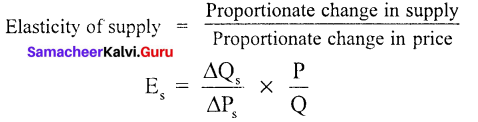Q – Supply, p – price, ∆ – change.

Question 32.
Bring out the Relationship among Total, Average and Marginal Products.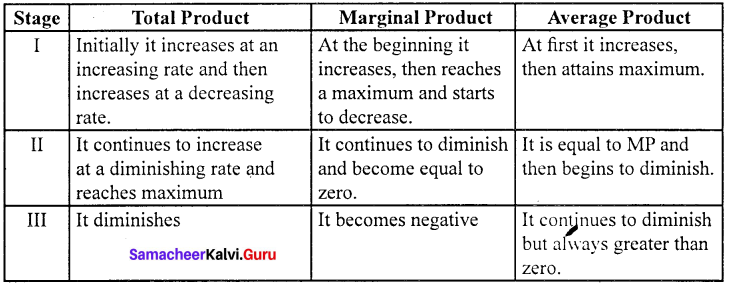Question 33.
Illustrate the concept of Producer’s Equilibrium.
Producer equilibrium is the situation where the producer maximizes his output. It is also known as the optimum combination of the factors of production.
(Eg.) Maximum output at minimum cost.
Producer attains equilibrium where the Iso-cost line is tangent to the Iso-quant.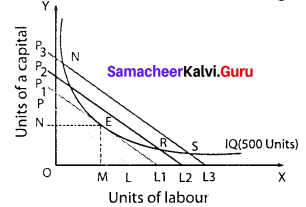In the figure profit of the firm is maximised at the point of equilibrium.
At the point of equilibrium slope of the Iso-cost line = Slope of Iso-product curve At the point E, the firm employs OM units of labour and ON units of capital which is the least cost combination.

Question 34.
State the Cobb – Douglas Production Function?
The Cobb – Douglas Production Function was developed by Charles W. Cobb and Paul H. Douglas, based on their empirical study of the American manufacturing industry. It is a linear homogeneous production function which implies that the factors of production can be substituted for one another up to a certain extent only.

The Cobb – Douglas production function can be expressed as follows.
Q = AL2K2
Where, Q = output; A = positive constant;
K = capital;
L = Labor α and β are positive fractions showing, the elasticity coefficients of outputs for the inputs labor and capital, respectively.
P = (1 – a) since a + p = 1. denoting constant returns to scale.
Factor intensity can be measured by the ratio β / α.

The sum of α + β shows the returns to scale.

1. (α + β) = 1, constant returns to scale.
2. (α + β) < 1, diminishing returns to scale.
3. (α + β) >1, increasing returns to scale.
• The production function explains that with the proportionate increase in the factors, the output also increases in the same proportion.
• Cobb – Douglas production function implies constant returns to scale.
Cobb – Douglas Production Function is a specific standard equation applied to describe how much output can be made with capital and labour inputs.

Part – D

Answer the following questions in about a page

Question 35.
Examine the Law of Variable Proportions with the help of a diagram.
According to G. Stigler, “As equal increments of one input are added, the inputs of other productive services being held constant, beyond a certain point the resulting increments of the product will decrease (ie) the marginal product will diminish”.
Assumptions:

1. Only one factor is variable.
2. All units of the variable factor are homogeneous
3. The product is measured in physical units.
4. There is no change in the state of technology and the price of the product.

Stage of production: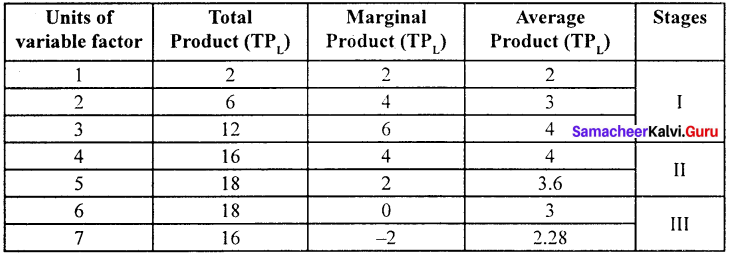Units of variable factor (labour) are employed along with other fixed factors. There are three stages of production.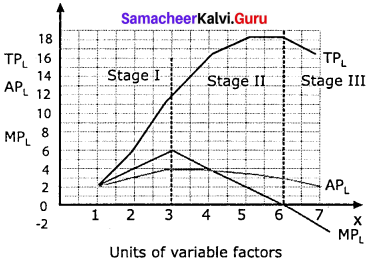Stage I:

1. In the first stage MPL increases upto third labourer and is higher than the average product.
2. So that total produt is increasing at an increasing rate.

Stage II:

1. MPL decreases up to sixth unit of labour where MPL curve intersects the x axis.
2. At fourth unit of labour MPL = APL
3. After this, MPL curve is lower than the APL. TPL increases at a decreasing rate.

Stage III:

1. The seventh unit of labour is marked by negative MPL the APL continues to fall but positive.
2. TPL declines with the employment of more units of labour.

Question 36.
List out the properties of Iso-quants with the help of diagrams.
Iso-quant:
“An Iso-quant is a curve showing all possible combinations of inputs physically capable of producing a given level of output” – Ferguson.

Properties of Iso-quant curve:
1. The Iso-quant curve has negative slope:

1. It slopes downwards from left to right indicating that the factors are substitutable.
2. In the diagram combination A refers to more of capital K5 and less of labour L2
3. As the producer moves to B, C and D more labour and less capital are used.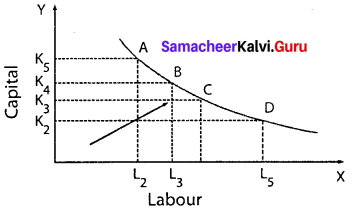2. Convex to the origin:

1. In production, the capital substituted by 1 unit of labour goes on decreasing. It is called diminishing marginal rate of technical substitution.
2. If the marginal rate of technical substitution diminishes the Iso-quants will be convex to the origin.
3. Constant MRTS (straight line) and increasing MRTS (concave).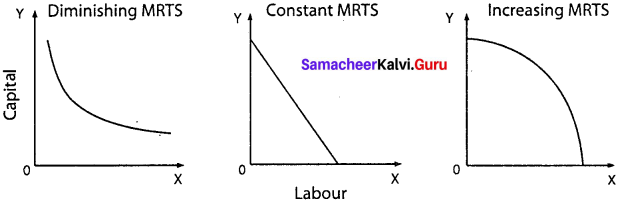3. Non-inter-section of Iso-Quant curves:

1. Point A lie on the Iso-quants IQ1 and IQ2
2. But the point C shows a higher output and point B shows a lower level of output IQ1
3. If C = A, B = A, then C – B. But C > B which is illogical.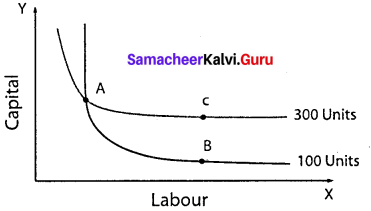4. An upper Iso-quant curve represents a higher level of output:

1. Higher IQs show higher outputs and lower IQs show lower output.
2. The arrow in the figure shows an increase in the output with a right and upward shift of an Iso-quant curve.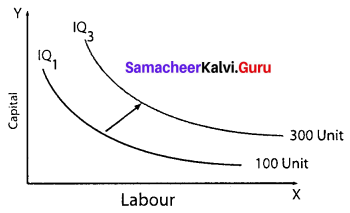5. Iso-quant curve does not touch either X axis or Y axis:
No Iso-quant curve touches the X – axis or Y – axis because in IQ(, only capital is used and in IQ2 only labour is used.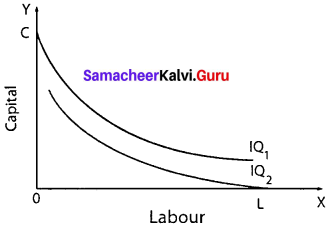Question 37.
Elucidate the Laws of Returns to Scale. Illustrate.
In the long-run all factors are variable. The laws of returns to scale explain the relationship between output and the scale of inputs in the long-run when all the inputs are increased in the same proportion.
Assumptions :

1. All the factors are variable except the organization.
2. There is no change in technology.
3. There is perfect competition in the market.
4. Outputs or returns are increased in physical quantities.

Three phases of returns to scale:
1. Increasing returns to scale:
If all inputs are increased by one percent, output increase by more than one percent.

2. Constant returns to scale:
In this case, if all inputs are increased by one percent, output increases by one percent.
Diagrammatic Illustration: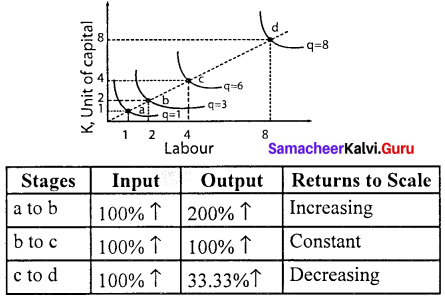In the diagram, the movement from point a to point b represents increasing returns to scale. Between these two points, input has doubled but the output was tripled.

The law of constant returns is implied by the movement from point b to point c. Between these two points inputs have doubled and output also has doubled.

Decreasing returns to scale are denoted by the movement from the point c to point d since doubling the factors from 4 units to 8 units produce less than the increase in inputs, that is by only 33.33%.

Question 38.
Explain the internal and external economies of scale.
The scale of production is an important factor affecting production. Every producer wishes to reduce the costs of production by using economies of scale.
Marshall classified economies into two.
1. Internal economies of scale: Internal economies refer to the reduction in the cost of production of the commodity. They are of various types.

• Technical economies: When the size and capital of the firm is large, up-to-date technologies can be used to improve the productivity of the firm. Here research and development strategies can be applied easily.
• Financial economies: Big firms can float shares in the market for capital expansion, while small firms cannot do so.
• Managerial economies: Large scale production facilitates specialization and delegation.
• Labour economies: Large scale production implies greater and minute division of labour. This leads to specialization which enhances the quality. This increases the productivity of the firm.
• Marketing economies: The producers can both buy raw-materials in bulk at a cheaper cost and can take the products to distant markets.
• Economies of survival: Product diversification is possible and it reduces the risk in production. Even if the market for one product collapses, the market for other commodities offsets it.

Question 39.
External economies of scale:
External economies of scale refer to changes in any factor outside the firm or industry causing an improvement in the production process.
Important external economies are:

1. Increased transport facilities.
2. Banking facilities
3. Development of townships
4. Development of information and communication

### Samacheer Kalvi 11th Economics Production Analysis Additional Questions and Answers

Question 1.
Production refers to …………………….
(a) Destruction of utility
(b) Creation of utilities
(c) Exchange value
(d) None
(b) Creation of utilities

Question 2.
_______ is a person who combines the factors of production in the production process to earn profit.
(a) Producer
(b) Entrepreneur
(c) Consumer
(d) Labours
(b) Entrepreneur

Question 3.
Division of labour is introduced by ………………………
(b) Marshall
(c) Schumpeter
(d) Hawley

Question 4.
Total product is
(a) TP = ∑AP
(b) TP = ∑MP
(c) TP = ∆N
(d) TP = TP / N
(b) TP = ∑MP

Question 5.
The Initial supply price of land is ……………………..
(a) Zero
(b) Greater than one
(c) Less than one
(d) Equal to one
(a) Zero

Question 6.
Cobb – Douglas production function depends on ______
(a) Land, Labour
(b) Land, Capital
(c) Labour, capital
(d) Labour, organisation
(c) Labour, capital

Question 7.
Who is the primary factor of production?
(a) Organizer
(b) Capital
(c) Machine
(d) Man
(a) Organizer

Question 8.
_______ is the man-made factor of production.
(a) Labour
(b) Capital
(c) Savings
(d) Land
(c) Savings

Question 9.
Technological relationship between inputs and output is called ……………………….
(a) Production function
(b) Technical function
(c) Capital function
(d) Organiser function
(a) Production function

Question 10.
In the production process _______ bears incertainity.
(a) Manager
(b) Labour
(c) Entrepreneur
(d) None
(c) Entrepreneur

Question 11.
α + β = 1 refers _______
(a) Increasing returns to scale
(c) Diminishing returns to scale
(d) None

Choose the correct statement

Question 1.
(a) Production refers to the creation of utility
(b) Production means the transformation of inputs into output
(c) Production creates economic well-being.
(d) The scale of production influences the revenue.
(d) The scale of production influences the revenue.

Question 2.
(a) An entrepreneur is a risk-taker – Walker.
(b) Capital consists of all kinds of wealth other than the free gift of nature -Marshall.
(c) An entrepreneur innovates – Schumpeter.
(d) An entrepreneur is an uncertainty bearer – Walker.
a. An entrepreneur is a risk-taker – Walker.

Match the following and choose the answer using the codes given below

Question 3.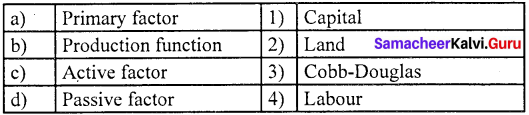a. 3 4 1 2
b. 1 2 3 4
c. 4 1 2 3
d. 2 1 3 4
(c) Active factor – (3) Cobb-Douglas

Question 4.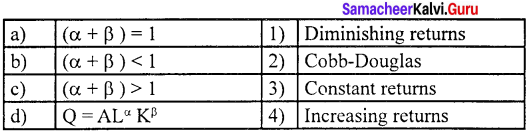a. 2 4 1 3
b. 1 2 3 4
c. 3 4 1 2
d. 4 1 2 3
(a) (α + β) =1 – (1) Diminishing returns.

Question 5.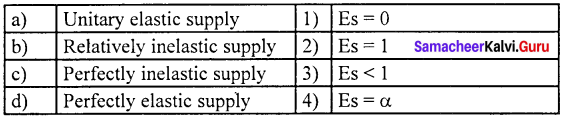a. 1 2 3 4
b. 4 1 2 3
c. 3 1 2 4
d. 2 3 1 4
(c) Perfectly inelastic supply = (3) Es < 1

Question 6.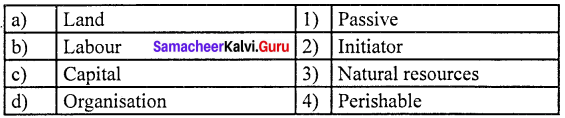a. 2 3 1 4
b. 3 4 1 2
c. 4 1 2 3
d. 3 4 2 1
(b) Labour = (2) Initiator

Choose the odd one out

Question 7.
(a) Land
(b) Labour
(c) Production
(d) Organisation
(c) Production

Question 8.
(a) Technical economics
(b) Financial economics
(c) Economics of survival
(d) Banking facility
(d) Banking facility

Choose the correct statement

Question 9.
(a) Iso-quant has a positive slope.
(b) Iso-quants never intersect each other
(c) Iso-quants are concave to the origin
(d) Iso-quant curve touch either X or Y-axis.
(b) Iso-quants never intersect each other

Question 10.
Cobb-Douglas production function.
(a) Implies constant returns to scale
(b) It considers all the factors of production.
(c) The elasticity of substitution between the factors is less than one.
(d) Is a non-linear homogeneous production function.
(a) Implies constant returns to scale

Analyze the reason for the following

Question 11.
Assertion (A) : Labour is the human input into the production process.
Reason (R) : Labour cannot be separated from the labourer.
a. Both (A) and (R) are true, (R) is the correct explanation of (A)
b. Both (A) and (R) are true, (R) is not the correct explanation of (A)
c. Both (A) and (R) are false.
d. (A) is true but, (R) is false.
b. Both (A) and (R) are true, (R) is not the correct explanation of (A)

Question 12.
Assertion (A) : Perishables have a low elasticity of supply.
Reason (R) : Perishables cannot be stored for a long time.
a. Both (A) and (R) are true, (R) is the correct explanation of (A)
b. Both (A) and (R) are true, (R) is not the correct explanation of (A)
c. (A) is true but (R) is false.
d. (A) is false but (R) is true.
a. Both (A) and (R) are true, (R) is the correct explanation of (A)

Choose the incorrect pair

Question 13.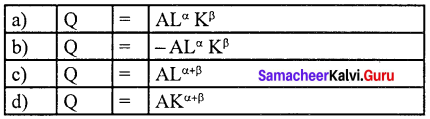(a) Q = ALα Kβ

Question 14.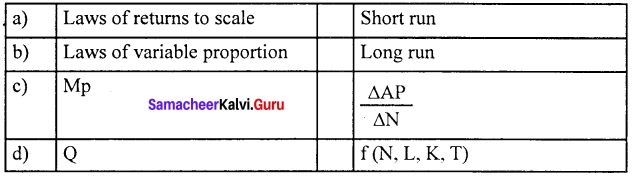(d) Q = f (N,L,K,T)

Fill in the blanks with the suitable option given below

Question 15.
Cobb-Douglas production function depends on _____
(a) Land, labour
(b) Land, capital
(c) Labour, capital
(d) Labour, organisation
(c) Labour, capital

Question 16.
Total product is _____
(a) TP = ∑AP
(b) TP = ∆N
(c) TP = ∑MP
(d) TP = $$\frac { TP }{ N }$$
(c) TP = ∑MP

Question 17.
In the production process __________ bears uncertainty.
(a) Manager
(b) Labour
(c) Worker
(d) Entrepreneur
(d) Entrepreneur

Choose the best option

Question 18.
is the man-made factor of production.
(a) Labour
(b) Capital
(c) Savings
(d) Land
(b) Capital

Question 19.
The labour exercised without expecting income is __________
(a) Service
(b) Physical labour
(c) Mental labour
(d) None of the above
(a) Service

Question 20.
Marginal product is ________
(a) MP = $$\frac { AP }{ N }$$
(b) MP = $$\frac { TP }{ N }$$
(c) MP = $$\frac { ∆AP }{ ∆N }$$
(d) MP = $$\frac { ∆TP }{ ∆N }$$
(d) MP = $$\frac { ∆TP }{ ∆N }$$

Part – B

Answer the following questions in one or two sentences

Question 1.
What are the characteristics of capital?

1. Capital is a man-made factor
2. Capital is mobile between places and persons
3. Capital is a passive factor of production
4. Capital’s supply is elastic
5. Capital’s demand is a derived demand
6. Capital is durable

Question 2.
What is the land?
Land means all gifts of nature owned and controlled by human beings which yield an income.

Question 3.
Explain the law of variable proportions assumptions?

1. The Law of Variable Proportions is based on the following assumptions.
2. Only one factor is variable while others are held constant.
3. All units of the variable factor are homogeneous.
4. The product is measured in physical units.
5. There is no change in the state of technology.
6. There is no change in the price of the product.

Question 4.
What is the total product?
Total product is the summation of marginal products.
TP = ∑MP

Question 5.
What is average product ?
Average product refers to the output per unit of the input.

Question 6.
What is diseconomies of scale ?
Diseconomies of scale are a disadvantage to a firm or industry or organisation. This necessarily increases the cost of production.

Question 7.
What are Iso-quants ?
An Iso-quant curve can be defined as the locus of points representing various combinations of two inputs yielding the same output.

Part – C

Answer the following questions in One Paragraph

Question 1.
State the characteristics of labour.

1. Labour is an active factor of production.
2. Labour may be manual or intellectual.
3. Labour is perishable.
4. Labour is inseparable from the labourer.
5. Labour is less mobile.
6. Labour is a means as well as an end.
7. Labour units are heterogeneous.
8. Labour has weak bargaining power.

Question 2.
Define Iso – quant, and Iso – quants Assumptions?
Definition of Iso – quant:
According to Ferguson, “An Iso – quant is a curve showing all possible combinations of inputs physically capable of producing a given level of output”.

Assumptions:

1. It is assumed that only two factors are used to produce a commodity.
2. Factors of production can be divided into small parts.
3. The technique of production is constant.
4. The substitution between the two factors is technically possible. That is, the production function is of “variable proportion” type rather than a fixed proportion.
5. Under the given technique, factors of production can be used with maximum efficiency.

Question 3.
Who is an entrepreneur?
An entrepreneur is a person who combines land, labour and capital in the production process to earn a profit. He not only runs the business but bears the risk of the business.

Question 4.
Differentiate the short period and long period.
The short-run is the period where some inputs are variable. Another feature is that firms do not enter or exit the industry. The long-run is the period where all the inputs are variable. It is featured by the entry of new firms and the exit of existing firms from the industry.

Question 5.
Name the classification of a production function.
Production function may be classified into two.

1. Short-run production function as illustrated by the law of variable proportions.
2. Long-run production function as explained by the laws of returns to scale.

Question 6.
What is an Iso-quant map?
An Iso-quant map has different Iso-quant curves representing the different combinations of factors of production, yielding the different levels of output. An Iso-quant map is a family of Iso-quants..

Part – D

Answer the following questions in about a page

Question 1.
What are the characteristics of labour?

1. Labour is the animate factor of production.
2. Labour is an active factor of production.
3. Labour implies several types: It may be manual [farmer] or intellectual [teacher, lawyer, etc]
4. Labour is perishable.
5. Labour is inseparable from the labourer.
6. Labour is less mobile between places and occupations.
7. Labour is a means as well as an end. It is both the cause of production and consumer of the product.
8. Labour units are heterogeneous.
9. Labour differs in ability.
10. Labour – supply determines its reward [wage]
11. Labour has weak bargaining power.

Question 2.
Explain the law of supply with a diagram.
1. Law of supply:
The Law of supply describes a direct relationship between the price of a good and the supply of that good.

2. Definition:
“Other things remaining the same, if the price of a commodity increases its quantity supplied increases and if the price of a commodity decreases, quantity supplied also decreases”.

3. Supply function:
Q = f (Px, Pr, Pr T, O, E)
Qs – Quantity supplied of x commodity
Px – Price of x commodity
Pr – Price of related goods
Pf – Price of factors of production.
T – Technology
O – Objective of the producer.
E – Expected price of the commodity

Assumptions:

1. There is no change in the prices of factors of production and capital goods.
2. Natural resources, technology, climate, political situations and tax policy remain unchanged.
3. Prices of substitutes are constant.

Supply schedule :
A supply schedule shows the different quantities of supply at different prices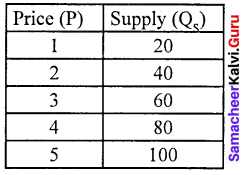Supply curve:
The quantum supplied is represented on X axis. And the price of the commodity is represent on the Y axis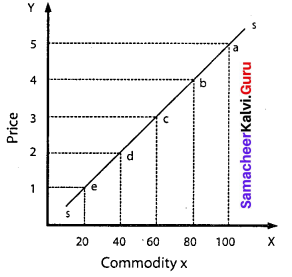As the price of the commodity increases, the quantum supplied of the commodity also increases. Thus the supply curve has a positive slope. The points such as e, d, c, b and a on the supply curve SS, represent various quantities of different prices.

Question 3.
Explain the factors determining supply.
1. Price of the commodity:
Higher the price larger the supply Price is the incentive for the producers and sellers to supply more.

2. Price of other commodities:
The supply of a commodity not only depends upon its price but also prices of other commodities. For instance if the price of commercial crops increases, the supply of food crops may decrease.

3. Price of factors:
When the input prices go up, this results in rise in cost and so supply will be affected.

4. Price expectations:
The expectation over future prices determines present supply.

5. Technology:
With advancement in technology, production level improves, average cost declines and as a result supply level increases.

6. Natural factors:
In agriculture, natural factors like a monsoon, climate, etc., play a vital role in determining the production level.

7. Discovery of new raw materials:
The discovery of cheaper and high-quality raw materials tends to increase the supply of the product.

8. Taxes and subsidies:
Subsidies encourage the producers to produce more whereas taxes kill the ability and willingness to produce more.

9. The objective of the firm:
When the goal of the firm is sales maximization or improving market share, the supply of the product is likely to be higher.

Question 4.
Explain the characteristics of labour.
According to Marshall, labour represents services provided by the factor labour, which helps in yielding an income to the owner of the labour-power.
Characteristics of labour:

1. Labour is the animate factor of production.
2. Labour is an active factor of production.
3. Labour implies several types it may be manual or intellectual.
4. Labour is perishable.
5. Labour is inseparable from the labourer.
6. Labour is less mobile between places and occupations.
7. Labour is a means as well as an end.
8. Labour units are heterogenous.
9. Labour-supply determines its reward.
10. Labour has weak bargaining power.

We wish the knowledge shared regarding Samacheer Kalvi for Class 11th Economics Solutions Chapter 3 Production Analysis Questions and Answers has been helpful to you. If you need any further help feel free to ask us and we will get back to you with the possible solution. Bookmark our site to avail the latest updates on different state boards solutions in split seconds.